4 1 Ratios Vocabulary ratio 4 1 Ratios

• Slides: 154 -1 Ratios Vocabulary ratio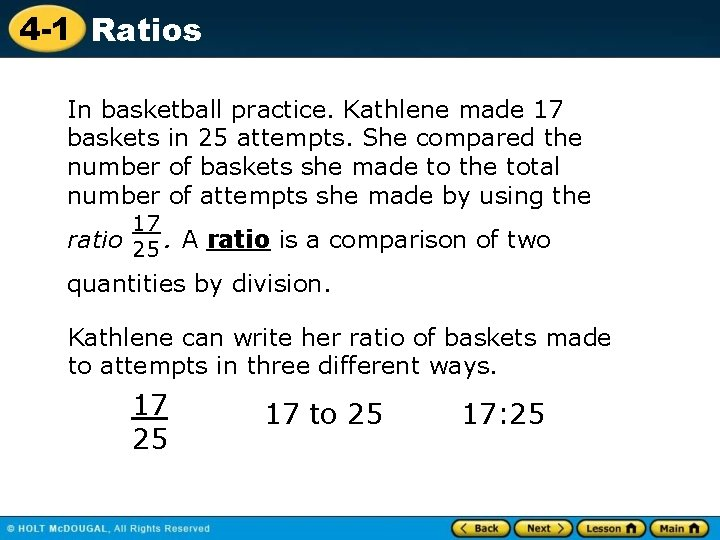4 -1 Ratios In basketball practice. Kathlene made 17 baskets in 25 attempts. She compared the number of baskets she made to the total number of attempts she made by using the 17 ratio 25. A ratio is a comparison of two quantities by division. Kathlene can write her ratio of baskets made to attempts in three different ways. 17 25 17 to 25 17: 254 -1 Ratios Additional Example 1: Writing Ratios Twenty students are asked to choose their favorite music category. Eight chose pop, seven chose hip hop, and five chose rock. Write each ratio in all three forms. A. rock to hip hop The ratio of rock to hip hop is 5 to 7, which can be written as follows: 5 , 5 to 7, 5: 7 7 B. hip hop to pop The ratio of hip hop to pop is 7 to 8, which can be written as follows: 7 , 7 to 8, 7: 8 8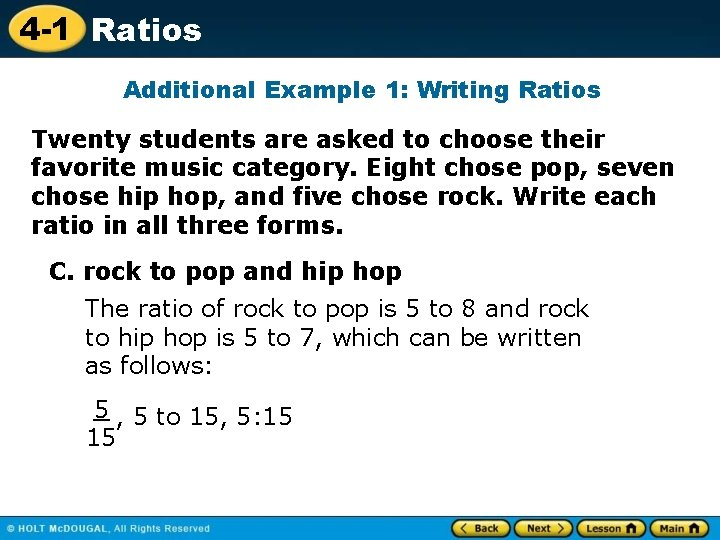4 -1 Ratios Additional Example 1: Writing Ratios Twenty students are asked to choose their favorite music category. Eight chose pop, seven chose hip hop, and five chose rock. Write each ratio in all three forms. C. rock to pop and hip hop The ratio of rock to pop is 5 to 8 and rock to hip hop is 5 to 7, which can be written as follows: 5 , 5 to 15, 5: 15 154 -1 Ratios Check It Out: Example 1 Nineteen students are asked to choose their favorite sport. Nine chose rock climbing, four chose kite surfing, and six chose snow boarding. Write each ratio in all three forms. A. snow boarding to rock climbing The ratio of snow boarding to rock climbing is 6 to 9, which can be written as follows: 6 , 6 to 9, 6: 9 9 B. kite surfing to snow boarding The ratio of kite surfing to snow boarding is 4 to 6, which can be written as follows: 4 , 4 to 6, 4: 6 6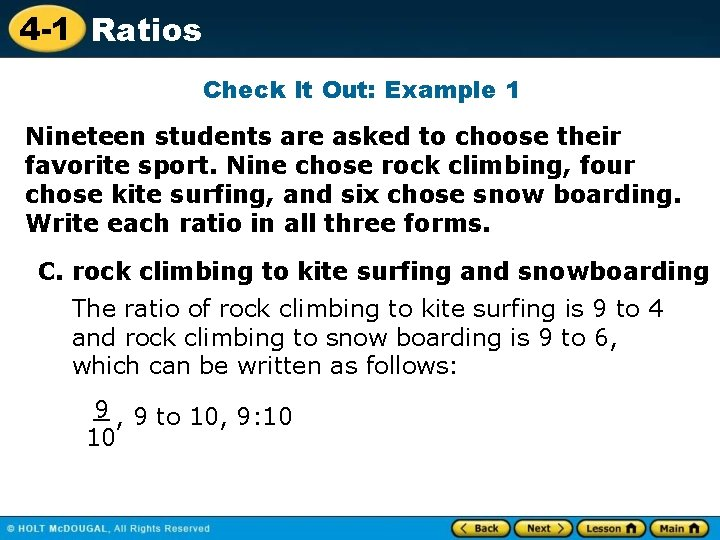4 -1 Ratios Check It Out: Example 1 Nineteen students are asked to choose their favorite sport. Nine chose rock climbing, four chose kite surfing, and six chose snow boarding. Write each ratio in all three forms. C. rock climbing to kite surfing and snowboarding The ratio of rock climbing to kite surfing is 9 to 4 and rock climbing to snow boarding is 9 to 6, which can be written as follows: 9 , 9 to 10, 9: 10 10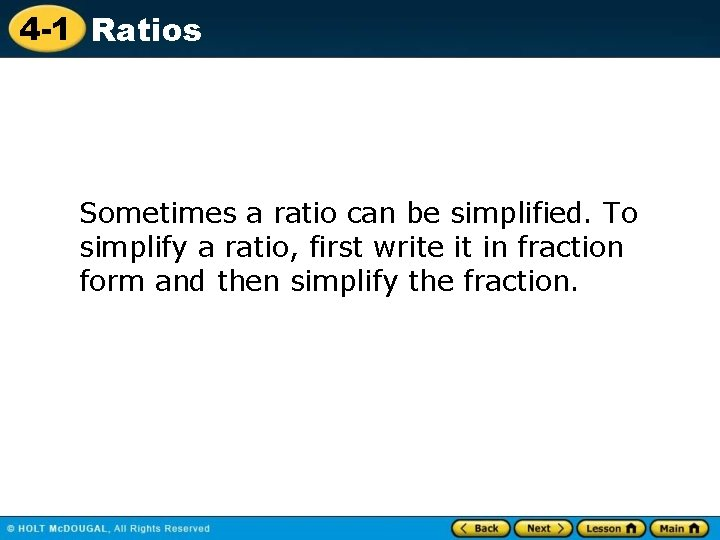4 -1 Ratios Sometimes a ratio can be simplified. To simplify a ratio, first write it in fraction form and then simplify the fraction.4 -1 Ratios Remember! A fraction is in simplest form when the GCF of the numerator and denominator is 1.4 -1 Ratios Additional Example 2: Writing Ratios in Simplest Form On average, most people can read about 600 words in 3 minutes. Write the ratio of words to minutes in all three forms. Write your answer in simplest form. words = 600 minute 3 Write the ratio as a fraction. words = 600 ÷ 3 minute 3÷ 3 Simplify. words = 200 minute 1 For every minute, there are 200 words read. The ratio of words to minutes is 200 to 1.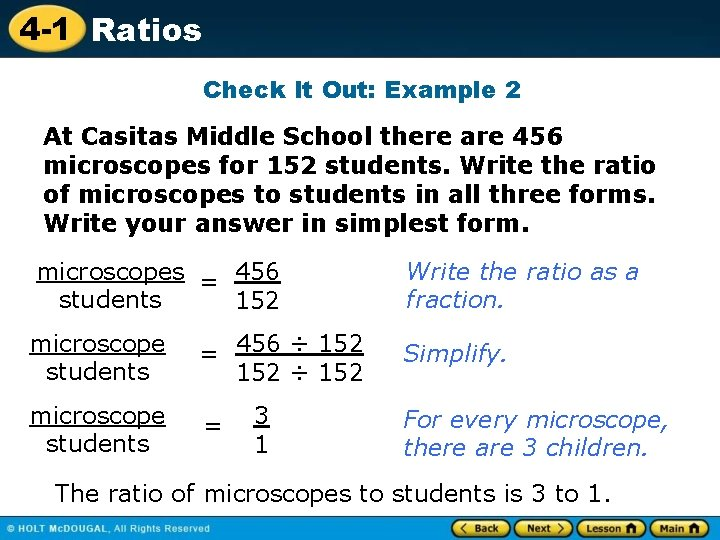4 -1 Ratios Check It Out: Example 2 At Casitas Middle School there are 456 microscopes for 152 students. Write the ratio of microscopes to students in all three forms. Write your answer in simplest form. microscopes = 456 students 152 Write the ratio as a fraction. microscope students = 456 ÷ 152 Simplify. microscope students = 3 1 For every microscope, there are 3 children. The ratio of microscopes to students is 3 to 1.4 -1 Ratios To compare ratios, write them as fractions with common denominators. Then compare the numerators.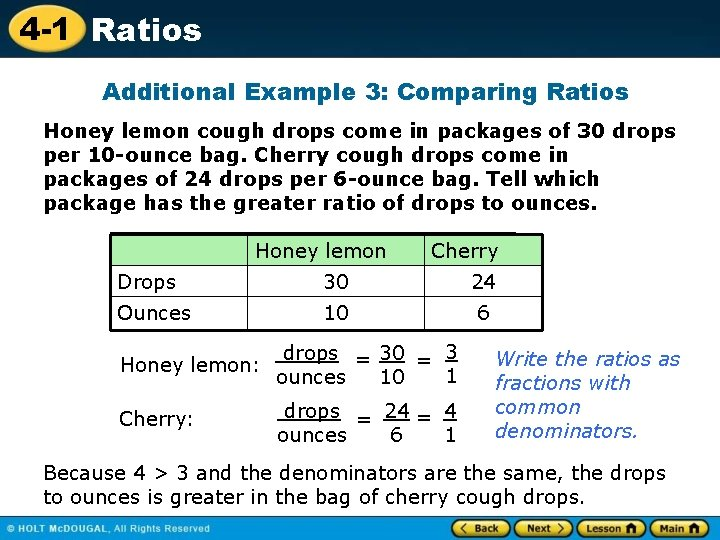4 -1 Ratios Additional Example 3: Comparing Ratios Honey lemon cough drops come in packages of 30 drops per 10 -ounce bag. Cherry cough drops come in packages of 24 drops per 6 -ounce bag. Tell which package has the greater ratio of drops to ounces. Honey lemon Cherry Drops 30 24 Ounces 10 6 drops = 30 = 3 Honey lemon: 1 ounces 10 Cherry: drops = 24 = 4 ounces 6 1 Write the ratios as fractions with common denominators. Because 4 > 3 and the denominators are the same, the drops to ounces is greater in the bag of cherry cough drops.4 -1 Ratios Check It Out: Example 3 Jelly beans come in small packages of 25 per 5 ounce package and large packages of 56 per 8 ounce package. Tell which package has the greater ratio of jelly beans to ounces. Large Small 56 25 8 5 Large: jelly beans ounces = 56 = 7 1 8 Small: jelly beans ounces = 25 = 5 5 1 Write the ratios as fractions with common denominators. Jelly beans Ounces Because 7 > 5 and the denominators are the same, jelly beans to ounces is greater in the small package.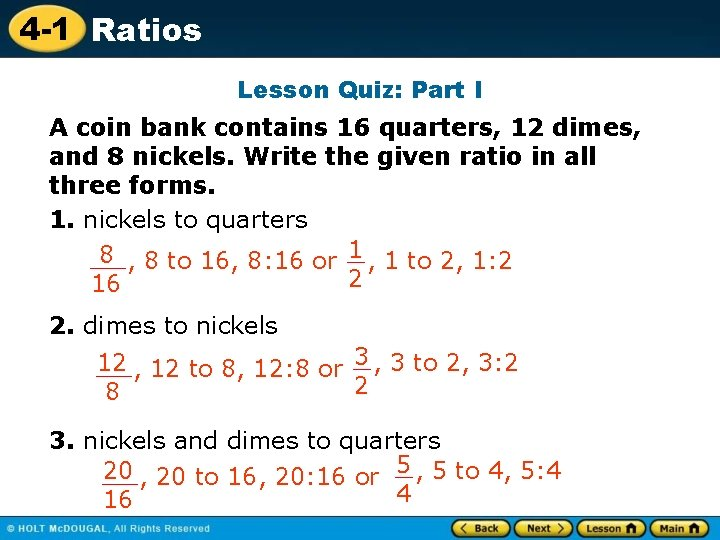4 -1 Ratios Lesson Quiz: Part I A coin bank contains 16 quarters, 12 dimes, and 8 nickels. Write the given ratio in all three forms. 1. nickels to quarters 8 , 8 to 16, 8: 16 or 1 , 1 to 2, 1: 2 2 16 2. dimes to nickels 12 , 12 to 8, 12: 8 or 3 , 3 to 2, 3: 2 2 8 3. nickels and dimes to quarters 20 , 20 to 16, 20: 16 or 5 , 5 to 4, 5: 4 4 164 -1 Ratios Lesson Quiz: Part II 4. There are 220 calories in 5 crackers. Write the ratio of calories to crackers in all three forms. Write your answers in simplest form. 44 calories , 44 to 1, 44: 1 1 cracker 5. On a school trip, bus 1 has 3 teachers and 14 students. Bus 2 has 4 teachers and 28 students. Which bus has the greater ratio of teachers to students? bus 1